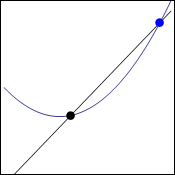# Quick point about equations and graphs

Hi,
mliae asked me to make some simpler post so she understands this post. So here it comes, she said that it was long since she used equations:

Well equation is something like this:

x=1

That is quite clear. Of course you can have very complicated equation with many “unknowns” which are usually noted as letters, x for example. All thats easy and it says that something (x) is equal to one, it has the value of 1.

We can manipulate these equations if we abide one rule: both sides (from left and right of equal sign) have to be manipulated. If we add one we have to do it on both sides:

x+1=1+1     —>    x+1=2

Easy. We can substitute in equations if we work with more:

a=b+42
2a+3=x

2(b+42)+3=x    (“a” was substitued by b+42 because that is what it equals to.)

Now in the post that is our concern we used this equation:To get here we have to use graph but I did not stop on that very much so I will go through it again.

Points on graph have two coordinates. This is because graph has 2 dimensions. These coordinates are usually called x and y and they are noted like this:

(x;y)

x says how much the point is to the left or right and y says how up or down (or closer/ further)

We had two points there on the graph:Now we will take the blue point as stacionary of course but since we are working in general and not with specific numbers, it should not matter.

So black point has coordinates x and y (x;y)

Since we want it to be general we will left it like this except the y. This is because we are going to derive functions and in those y=f(x) which means that y coordinate of the point is f(x). That is the notation that is used. We are working with the function f that gave coordinate y to our black point.

black_point(x;f(x))
blue_point(x+h;f(x+h))

The h should be clear from last time. It is the distance from the black point. Then when you insert this into the “slope” equation which I talked about here, you will get what you want.

Dragallur

Feel free to ask for more clarification.

# Explaining equations easy way

Hi,
today I want to talk about simple physical equations and how you can check if they are right or not.

Lets start with the equation of distance in the most simple way.  How do you find this formula anyway? Well we want to know how distance traveled is calculated.

s=…

Now on the right side there should be something that corresponds to distance. There has to be time because we are talking about traveling in some time.

s=t…

Distance also depends on how fast you are traveling, which means velocity has to be there. Now if both time and velocity are bigger you will get also bigger distance so there will be multiplication between them.

s=v*t

From this we can conclude that:

v=s/t     this makes sense because velocity will be big when you travel for short time great distance.

t=s/v     this too makes sense because time will be great when you will go slowly great distance.

This is all intuitive, you do not remember any equations because those you can always get just from pure logic.

I can follow with the equation of inertia:

p=…

Inertia describes basicly how hard it is to stop something. Just from personal experience, when is it hard to stop something? When it is heavy and when it is fast.

p=m*v

What units will inertia have? Well you can always compare only same units so the units that come up from “m*v” must be the units of inertia.

p=m*s/t (now here we have the basic units)

p=kilograms*meters/seconds

The unit of inertia is kilogram on meter per second!

Those equations that have only two parts can be made really fast this way if you do not remember it exactly.

There is this important part with the same units. When you do some problem in physics you usually first just take all the letters and try to combine them until you get the final “sentence” and then you enter all your values. This is very effective and it is good to check you final equation, look at this for example:

P=m*g*s/t

Here it would be hard to imagine if it makes sense like we did with the formula for distance but we can get insert all the units and check if the right side is the unit for P – performance. (here m is mass, g is gravitational acceleration, s is distance, t is time). We know that the unit of performance is watt and watt is: kg*meter^2/second^3

So now for the equation we insert the values:

P=(mass*(meter/second^2)*meter)/second

P=kg*meter^2/second^3

Now we know that we are right even though we maybe started with P=W/t (W is work) and we were not sure if the conversion was right. The units correspond to what we know about performance.

Dragallur